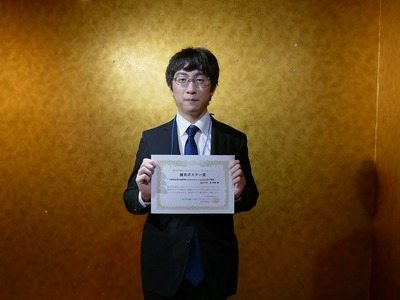'工学部/工学系研究科　トピックス' blog (64135253074) of hub id 20511701

# Yuki Mori (Major MSc student), Department of Nuclear Engineering and Management, received Best Poster Award from the The 23rd SCEJ Symposium on Fluidization & Particle Processing

On 7th December 2017, Mr. Yuki Mori (Major MSc student), Department of Nuclear Engineering and Management, received Best Poster Award from the The 23rd SCEJ Symposium on Fluidization & Particle Processing.
It was given for the superior presentation at poster session at this symposium.Numerical simulations of fluidized bed are often performed for analyzing this behavior. In numerical simulation, DEM-CFD method, one of the Eulerian-Lagrangian approach, is often used. Although this method is well established, it has a couple of problem, such as substantial limit of number of calculated particles, and difficulty of calculating gas-solid flow in arbitrary shaped-wall effectively. For these problems, numerical simulation of large-scale engineering fluidized bed was very difficult.
Then, to solve these problems, we developed new numerical simulation method. In this method, coarse grain model(CGM) is introduced. In this model, a group of particles are represented by a coarse grain particle, then this model enabled us to use enormous number of original particles. Besides, Advanced DEM-CFD method are also introduced for calculated boundary wall of solid and fluid phase. However, by using these methods, we couldn’t calculate large-scale engineering fluidized bed, because drag force term are very big in dense place, and tend to divergence calculation. Thus, we developed new implicit method for drag force term. It enables us to execute numerical simulation stably.
Finally, in order to validate developed method, we performed numerical simulation and experiment of fluidized bed under comparable conditions.